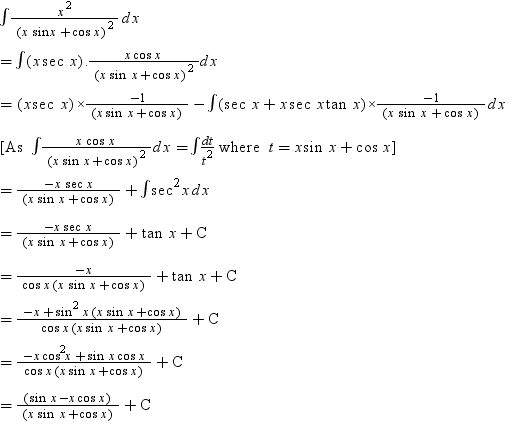# Integration of x^2/(xsinx+cosx)^2

• JasonHathaway

#### JasonHathaway

Hi everyone,

First of all, this isn't really a "homework", I've completed my calculus course and I'm just curious about this problem.

## Homework Statement

$\int\frac{x^{2}}{(xsinx+cosx)^{2}} dx$

## Homework Equations

Trigonometric substitutions, integration by parts maybe?

## The Attempt at a Solution

This is a solved problem.How does $\int\frac{x^{2}}{(xsinx+cosx)^{2}} dx$ become $\int xsecx \frac{xcosx}{(xsinx+cosx)^{2}} dx$?

Just because $$sec(x)=\frac{1}{cos(x)}$$

Did it multiply the numerator and denominator by $\frac{cosx}{cosx}$, which is $cosx secx$, and then both of $cosx$ and $secx$ took one "x" from the original numerator?

JasonHathaway said:
Did it multiply the numerator and denominator by $\frac{cosx}{cosx}$, which is $cosx secx$, and then both of $cosx$ and $secx$ took one "x" from the original numerator?
Yes.

In other words, ##\ \cos(x)\cdot\sec(x) = 1 \ .##